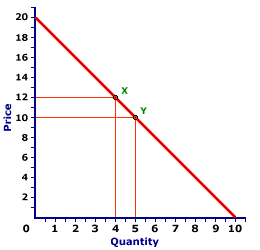Tuesday  February 7, 2023
 AmosWEB means Economics with a Touch of Whimsy!JOB VACANCY RATE: A simple little ratio of the number of job vacancies in our economy to the sum of employment and job vacancies. In essence, this measures the fraction of jobs in the economy that are open, but haven't been filled. To be included as an officially vacant job, employers must be actively searching to fill it with a warm body, by advertising in the paper, contacting employment offices, etc. Like the more common unemployment rate, the job vacancy rate is a useful indicator of the business cycle. When the economy is booming, the job vacancy rate is likely to be relatively high. A low rate signals a recession.ENDPOINT ELASTICITY FORMULA:

A simple technique for calculating the coefficient of elasticity by estimating the elasticity for discrete changes in two variables using the initial values of each. The distinguishing characteristic of this formula is that percentage changes are calculated based on the initial values of each variable. An alternative technique is the midpoint elasticity formula.
The endpoint elasticity formula is a simple, first-approximation, method of calculating elasticity, especially the price elasticity of demand, price elasticity of supply, income elasticity of demand, and cross elasticity and demand. This formula is most often used at the introductory level of economic instruction.

### The Formula

The endpoint elasticity formula for calculating the response of changes in B to changes in A is given as:

 endpointelasticity = (B2 - B1)B1 ÷ (A2 - A1)A1
The first term on the right-hand side of the equation is the percentage change in variable B. The second term is the percentage change in variable A. The individual items are interpreted as this: A1 is the initial value of A before any changes, A2 is the ending value after A changes, B1 is the initial value of B before any changes, and B2 is the ending value after B changes.

The numerator of each term on the right-hand side of the equation [(A2 - A1) and (B2 - B1)] is the discrete change in A and B, respectively. The denominator of each term, A1 and B1, is the base value from which the percentage change is calculated.

### An Simple Example

A Standard Demand CurveThe time has come for a numerical example to illustrate. The demand curve to the right should help. Suppose the demand price of Wacky Willy Stuffed Amigos (those cute and cuddly armadillos and tarantulas) INCREASES from \$10 to \$12. This price increase causes the quantity demanded to decrease from 5 to 4 Stuffed Amigos. Using the endpoint elasticity formula, the price increases by 20 percent, a \$2 increase from a \$10 initial price. This method also results in a 20 percent decrease in the quantity demanded, a decline of 1 Stuffed Amigo from an initial value of 5. This seems relatively simple and straightforward. The price elasticity of demand using the endpoints elasticity formula is thus equal to 1.

Or is it?

### An Endpoint Problem

The problem with the endpoints elasticity formula for demand elasticity is that the coefficient of elasticity depends on whether price increases or decreases. This is demonstrated by asking the question: What is the coefficient of elasticity for this same range of the demand curve, if the price DECREASES from \$12 to \$10 causing the quantity demanded to increase from 4 to 5? In this case, the change in price is 16.7 percent, a \$2 decrease from a \$12 initial price, and the change in quantity is 25, an increase of 1 Stuffed Amigo from an initial value of 4. This results in a coefficient of elasticity of 1.5.

Over the same segment of the demand curve, a price INCREASE indicates a different coefficient of elasticity than does a price DECREASE. This can become confusing.

This problem is prevented by using an alternative method of calculating the coefficient of elasticity, the midpoint elasticity formula. This alternative uses the average of the beginning and ending values of each variable (that is, the midpoint) as the base value for calculating percentage changes. If the discrete changes are relatively small, then the two methods are approximately the same.

 <= EMPLOYMENT RATE ENERGY PRICES, AGGREGATE SUPPLY DETERMINANT =>Recommended Citation:

ENDPOINT ELASTICITY FORMULA, AmosWEB Encyclonomic WEB*pedia, http://www.AmosWEB.com, AmosWEB LLC, 2000-2023. [Accessed: February 7, 2023].

Check Out These Related Terms...

Or For A Little Background...

And For Further Study...
Search Again?BLUE PLACIDOLA[What's This?] Today, you are likely to spend a great deal of time looking for the new strip mall out on the highway hoping to buy either a rechargeable battery for your computer or shoe laces for your snow boots. Be on the lookout for defective microphones.Your Complete ScopeOne of the largest markets for gold in the United States is the manufacturing of class rings."Everyone's got it in him, if he'll only make up his mind and stick at it. None of us is born with a stop-valve on his powers or with a set limit to his capacities. There's no limit possible to the expansion of each one of us."-- Charles M. SchwabG7Group of SevenA PEDestrian's Guide Xtra CreditTell us what you think about AmosWEB. Like what you see? Have suggestions for improvements? Let us know. Click the User Feedback link.| | | | | | | | | | |
| | | |

Thanks for visiting AmosWEB